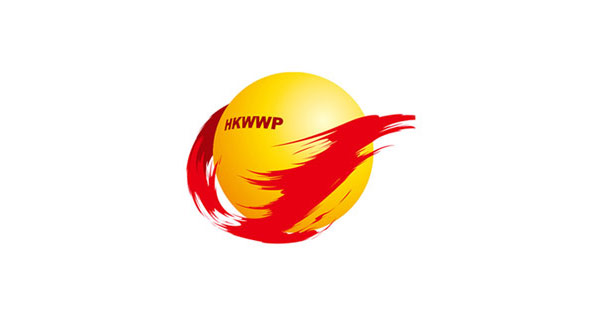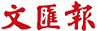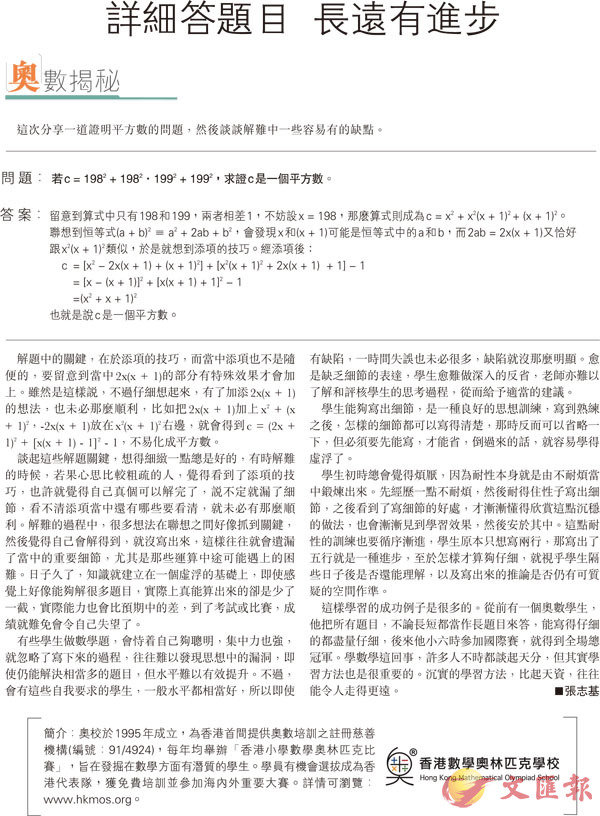首頁 > 文匯報 > 教育 > 正文

# 【奧數揭秘】詳細答題目 長遠有進步

2019-11-13c= [x2 - 2x(x + 1) + (x + 1)2] + [x2(x + 1)2 + 2x(x + 1) + 1] - 1

= [x - (x + 1)]2 + [x(x + 1) + 1]2 - 1

=(x2 + x + 1)2

■香港數學奧林匹克學校